# Excel LOOKUP function with formula examples

The tutorial explains the vector and array forms of the Excel LOOKUP function and demonstrates typical and non-trivial uses of LOOKUP in Excel with formula examples.

One of the most frequent questions that every Excel user asks once in a while is this: "How do I look up a value on one sheet and pull a matching value to another sheet?". Of course, there can be many variations of the basic scenario: you may be looking for the closest match rather than exact match, you may want to search vertically in a column or horizontally in a row, evaluate one or multiple criteria, etc. However, the essence is the same - you need to know how to look up in Excel.

Microsoft Excel provides a handful of different ways to do lookup. To begin with, let's learn a function that is designed to handle the simplest cases of vertical and horizontal lookup. As you can easily guess, I am talking about the LOOKUP function.

## Excel LOOKUP function - syntax and uses

At the most basic level, the LOOKUP function in Excel searches a value in one column or row and returns a matching value from the same position in another column or row.

There are two forms of LOOKUP in Excel: Vector and Array. Each form is explained individually below.

## Excel LOOKUP function - vector form

In this context, a vector refers to a one-column or one-row range. Consequently, you use the vector form of LOOKUP to search one row or one column of data for a specified value, and pull a value from the same position in another row or column.

The syntax of the vector Lookup is as follows:

LOOKUP(lookup_value, lookup_vector, [result_vector])

Where:

• Lookup_value (required) - a value to search for. It can be a number, text, logical value of TRUE or FALSE, or a reference to a cell containing the lookup value.
• Lookup_vector (required) - one-row or one-column range to be searched. It must be sorted in ascending order.
• Result_vector (optional) - one-row or one-column range from which you want to return the result - a value in the same position as the lookup value. Result_vector must be the same size as lookup_range. If omitted, the result is returned from lookup_vector.

The following examples demonstrate two simple Lookup formulas in action.

#### Vertical Lookup formula - search in one-column range

Let's say, you have a list of sellers in column D (D2:D5) and the products they sold in column E (E2:E5). You are creating a dashboard where your users will enter the seller's name in B2 and you need a formula that would pull a corresponding product in B3. The task can be easily accomplished with this formula:

`=LOOKUP(B2,D2:D5,E2:E5)`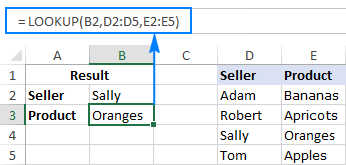To better understand the arguments, please have a look at this screenshot: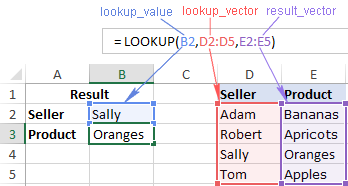#### Horizontal Lookup formula - search in one-row range

If your source data has a horizontal layout, i.e. the entries reside in rows rather than columns, then supply a one-row range in the lookup_vector and result_vector arguments, like this:

`=LOOKUP(B2,E1:H1,E2:H2)`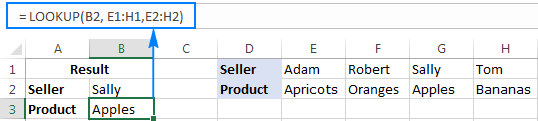In the second part of this tutorial, you will find a few more Excel Lookup examples that solve more complex tasks. In the meantime, please remember the following simple facts that will help you bypass possible pitfalls and prevent common errors.

### 5 things you should know about vector form of Excel LOOKUP

1. Values in lookup_vector should be sorted in ascending order, i.e. from smallest to largest or from A to Z, otherwise your Excel Lookup formula may return an error or incorrect result. If you need to do lookup on unsorted data, then use either INDEX MATCH or OFFSET MATCH.
2. Lookup_vector and result_vector must be a one-row or one-column range of the same size.
3. The LOOKUP function in Excel is case-insensitive, it does not differentiate uppercase and lowercase text.
4. Excel LOOKUP works based on approximate match. More precisely, a Lookup formula searches for exact match first. If it cannot find the lookup value exactly, it looks up the next smallest value, i.e. the largest value in lookup_vector that is less than or equal to lookup_value.

For example, if your lookup value is "5", the formula will search it first. If "5" is not found, it will search "4". If "4" is not found, it will search "3", and so on.

5. If lookup_value is smaller than the smallest value in lookup_vector, Excel LOOKUP returns the #N/A error.

## Excel LOOKUP function - array form

The array form of the LOOKUP function searches the specified value in the first column or row of the array and retrieves a value from the same position in the last column or row of the array.

The array Lookup has 2 arguments, both of which are required:

LOOKUP(lookup_value, array)

Where:

• Lookup_value - a value to search for in an array.
• Array - a range of cells where you want to search for the lookup value. The values in the first column or row of the array (depending on whether you do V-lookup or H-lookup) must be sorted in ascending order. Uppercase and lowercase characters are deemed equivalent.

For example, with the seller names located in the first column of the array (column A) and order dates in the last column of the array (column C), you can use the following formula to search the name and pull the matching date:

`=LOOKUP(B2,D2:F5)`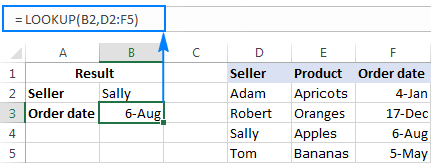Note. The array form of the Excel LOOKUP function should not be confused with Excel array formulas. Although it operates on arrays, LOOKUP is still a regular formula, which is completed in the usual way by pressing the Enter key.

### 4 things you should know about array form of Excel LOOKUP

1. If array has more rows than columns or the same number of columns and rows, a Lookup formula searches in the first column (horizontal lookup).
2. If array has more columns than rows, Excel LOOKUP searches in the first row (vertical lookup).
3. If a formula can't find the lookup value, it uses the largest value in the array that is less than or equal to lookup_value.
4. If the lookup value is smaller than the smallest value in the first column or row of the array (depending on the array dimensions), a Lookup formula returns the #N/A error.

Important note! The functionality of the Excel LOOKUP array form is limited and we don't recommend using it. Instead, you can use the VLOOKUP or HLOOKUP function, which are the improved versions to do vertical and horizontal lookup, respectively.

## How to use LOOKUP function in Excel - formula examples

Although there exist more powerful functions to look up and match in Excel (which is the subject of our next tutorial), LOOKUP comes in handy in many situations, and the following examples demonstrate a couple of non-trivial uses. Please note, all of the below formulas use the vector form of Excel LOOKUP.

### Look up a value in the last non-blank cell in a column

If you have a column of dynamically populated data, you may want to pick the most recently added entry, i.e. get the last non-empty cell in a column. For this, use this generic formula:

LOOKUP(2, 1/(column<>""), column)

In the above formula, all arguments except for the column reference are constants. So, to retrieve the last value in a specific column, you just need to supply the corresponding column reference. For example, to extract the value of the last non-blank cell in column A, use this formula:

`=LOOKUP(2, 1/(A:A<>""), A:A)`

To get the last value from other columns, modify the column references like shown in the screenshot below - first reference is the column to be checked for blank/non-blank cells, and the second reference is the column to return the value from: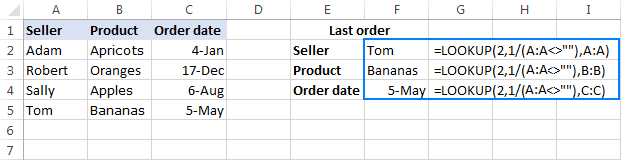#### How this formula works

In the lookup_value argument, you supply 2 or any other number greater than 1 (in a moment, you will understand why).

In the lookup_vector argument, you put this expression: 1/(A:A<>"")

• First, you perform the logical operation A:A<>"" that compares each cell in column A with an empty string and returns TRUE for empty cells and FALSE for non-empty cells. In the above example, the formula in F2 returns this array: {TRUE;TRUE;TRUE;TRUE;FALSE...}
• Then, you divide the number 1 by each element of the above array. With TRUE equating to 1 and FALSE equating to 0, you get a new array consisting of 1's and #DIV/0! errors (the result of dividing by 0), and this array is used as lookup_vector. In this example, it's {1;1;1;1;#DIV/0!...}

Now, how it comes that the formula returns the last non-empty value in a column, given that lookup_value does not match any element of lookup_vector? The key to understanding the logic is that Excel LOOKUP searches with approximate match, i.e. when the exact lookup value is not found, it matches the next biggest value in lookup_vector that is smaller than lookup_value. In our case, lookup_value is 2 and the largest value in lookup_vector is 1, so LOOKUP matches the last 1 in the array, which is the last non-empty cell!

In the result_vector argument, you reference the column from which you want to return a value, and your Lookup formula will fetch the value in the same position as the lookup value.

Tip. If you wish to get the number of the row holding the last value, then use the ROW function to retrieve it. For example: `=LOOKUP(2,1/(A:A<>""),ROW(A:A))`

### Look up a value in the last non-blank cell in a row

If your source data is laid out in rows rather than columns, you can get the value of the last non-empty cell using this formula:

LOOKUP(2, 1/(row<>""), row)

In fact, this formula is nothing else but a slight modification of the previous formula, with the only difference that you use the row reference instead of the column reference.

For example, to get the value of the last non-empty cell in row 1, use this formula:

`=LOOKUP(2, 1/(1:1<>""), 1:1)`

The following screenshot shows the result: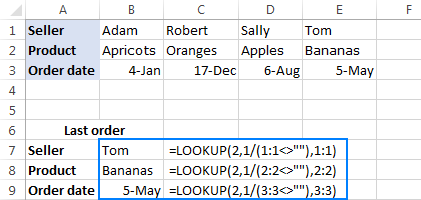### Get a value associated with the last entry in a row

With just a little creativity, the above formula can easily be customized for solving other similar tasks. For instance, it can be used to get a value associated with the last instance of a specific value in a row. This may sound a bit obscure, but the following example will make things easier to understand.

Assuming you have a summary table where column A contains the seller names and subsequent columns contain data of some kind for each month. In this example, a cell contain "yes" if a given seller has closed at least one deal in a given month. Our goal is to get a month associated with the last "yes" entry in a row.

The task can be solved by using the following LOOKUP formula:

`=LOOKUP(2, 1/(B2:H2="yes"), \$B\$1:\$H\$1)`

The formula's logic is basically the same as described in the first example. The difference is that you use the "equal to" operator ("=") instead of "not equal to" ("<>") and operate on rows instead of columns.

The following screenshot demonstrates a result: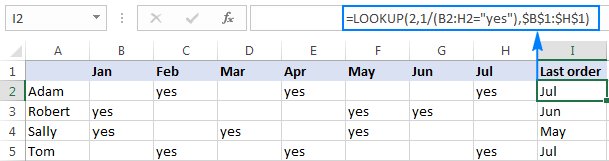### Lookup as alternative to nested IFs

In all of the Lookup formulas we've discussed so far, the lookup_vector and result_vector arguments were represented by range references. However, the syntax of the Excel LOOKUP function allows supplying the vectors in the form of a vertical array constant, which enables you to replicate the functionality of nested IF with a more compact and easy-to-read formula.

Let's say, you have a list of abbreviations in column A and you want to replace them with full names, where "C" stands for "Completed", "D" is "Development, and "T" is "Testing". The task can be accomplished with the following nested IF function:

`=IF(A2="c", "Completed", IF(A2="d", "Development", IF(A2="t", "Testing", "")))`

Or, by using this Lookup formula:

`=LOOKUP(A2, {"c";"d";"t"}, {"Completed";"Development";"Testing"})`

As shown in the screenshot below, both formulas yield identical results: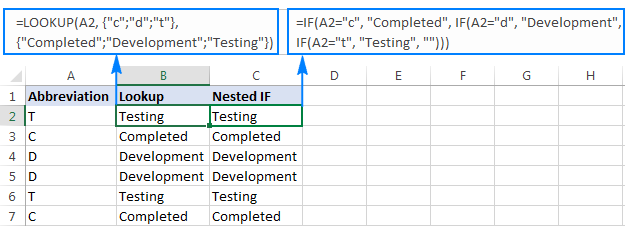Note. For an Excel Lookup formula to work correctly, the values in lookup_array should be sorted from A to Z or from smallest to largest.

If you are pulling values from a lookup table, then you can embed a Vlookup function in the lookup_value argument to retrieve a match.

Assuming the lookup value is in cell E2, the lookup table is A2:C7, and the column of interest ("Status") is the 3rd column in the lookup table, the following formula does the job:

`=LOOKUP(VLOOKUP(E2, \$A\$2:\$C\$7, 3, FALSE), {"c";"d";"t"}, {"Completed";"Development";"Testing"})`

As demonstrated in the screenshot below, the formula retrieves the project status from the lookup table and replaces an abbreviation with the corresponding word: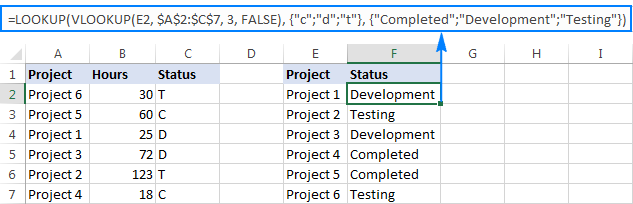Tip. If you are using Excel 2016 as part of an Office 365 subscription, you can use the SWITCH function for similar purposes.

I hope these examples have shed some light on how the LOOKUP function works. To better understand the formulas, you are welcome to download these Excel Lookup examples. In the next tutorial, we will discuss a few other ways to do lookup in Excel and explain which Lookup formula is best to be used in which situation. I thank you for reading and hope to see you on our blog next week!

## You may also be interested in

### 74 comments to "Excel LOOKUP function with formula examples"

1.WILLIAM O'DONNELL says:

I am looking to pull data from one tab into a consolidated table, The tab I am pulling from matches a customer column and date column and needs to pull from a column that may have multiple cells to give a total. What would be the best method to accomplish this in excel.

•Alexander Trifuntov (Ablebits Team) says:

Hi!
Unfortunately, without seeing your data it is difficult to give you any advice. If you have a specific question about the operation of a function or formula, I will try to answer it.

2.Iqbal Hadi says:

Hi Svetlana,

Good morning!

I have a duty roster of nurses for the month of Feb. Below here is a sample. I want a formula that can give me the last two days' duty. (E.g EN). Each month we have days ending. I have tried =CONCATENATE but it's not working every month.

E E N N O O M M E E N N O O M M E E N N N O O M M E E N

•Alexander Trifuntov (Ablebits Team) says:

Hi!
I’m sorry but your task is not entirely clear to me. Could you please describe it in more detail? What result do you want to get?

3.Sachin says:

Sir, where can i attache a spread sheet?

Sr. No Code Name City
1 123 abcd xyz
456 efgh abc

2 789 fgju obc
987 tyqw cnn

above data is in sheet one

in another sheet i wanna select only sr no. , for that i m using vlookup but here main problem is the Sr No is using for both upper row and in other sheet i need both row data by selecting sr. no.

for example if i select sr. no. 2 than it will have to show me data of sr. no. 2 which is as below

Code Name City
789 fgju obc
987 tyqw cnn

pls provide me an email address to mail you my problem and data spreadsheet

4.SACHIN says:

Sr. Code Name Department Branch DOJ CITY
1 125 ABCD A AS 01-02-2020 KOLKATA
521 BCDE B AC 01-04-2021 MUMBAI
688 CDEF E AN 01-09-2020 DELHI
420 EFGH L AM 15-08-2020 AHMEDABAD
297 FGHI P AO 01-07-2020 AGRA
108 GHIJ W AP 01-04-2020 GOA
2 735 XYZ M AC 15-08-2020
926 YZA I AN 01-07-2020
509 ZAB K AM 01-04-2020
645 ABC D AS 15-08-2020
135 BCD Q AC 01-07-2020
900 CDE E AL 01-04-2020

I WANNA MANGE THIS DATA TO ANOTHER SHEET BY SCROLLING A MACRO BUTTON SELECTING ONLY 1ST COLUMN SR NO AND FILL ALL DATA WITHIN CODE 125 TO CITY GOA

CAN ANY ONE HELP ME WITH VLOOKUP OR HLOOKUP OR XLOOKUP FORMULAS
I CAN SHARE EXCEL SHEET TO EXPLAIN MORE CLEARLY

5.Luzs says:

Thanks

6.Luzs says:

Date Activities Planned Actual Shift
2021/06/01 SD 5 2 Day - Shift
2021/06/01 FD 4 2 Day - Shift
2021/06/02 FD 4 2 Day - Shift
2021/06/03 FD 4 2 Day - Shift
2021/06/04 FD 4 2 Day - Shift
2021/06/07 SD 5 2 Day - Shift
2021/06/08 SD 5 2 Day - Shift

sheet 2

7.Luzs says:

I need to look at the current date on sheet 1, then look at sheet 2 same date, shift and activity, then return the actual

8.Luzs says:

I am trying to pull data from sheet 2 to sheet 1 which is a report.

sheet 2
A: date
B: Activities are (SD, FD and DD)
C: Target
D: Actual
E: Shifts: (Day, Afternoon and Night)

so I want to pull the actuals to the report sheet 1

All the 3 activities happens in all 3 shifts

9.Luzs says:

is there anything wrong with this formula? is just not giving me what is suppose to give me

=VLOOKUP((('North Section'!J:J=(TODAY()-1))*('North Section'!K:K="SD")*('North Section'!N:N="Day - Shift "));Flat_file__2;13;FALSE)

10.Caroline says:

I need a Lookup formula to do this:
Lookup if B2 = "CN" and C27 = "A" then get a predefined value.

So if B2 = "CN" and C27 = "A" then show £1
OR if B2 = "CN" and C27 = "B" then show £2
OR if B2 = "CN" and C27 = "C" then show £3
OR if B2 = "CN" and C27 = "D" then show £4
So if B2 = "PI" and C27 = "A" then show £1.50
OR if B2 = "PI" and C27 = "B" then show £2.50
OR if B2 = "PI" and C27 = "C" then show £3.50
OR if B2 = "PI" and C27 = "D" then show £4.50

Can anyone help?

•Alexander Trifuntov (Ablebits Team) says:

Hello!

=IF(B2="CN",1,0)*MATCH(C27,{"A","B","C","D"},0) + IF(B2="PI",1,0)*(MATCH(C27,{"A","B","C","D"},0)+0.5)

Hope this is what you need.

•Caroline says:

Your formula does work (thank you) but i need differing cell referrals for a then b then c etc

Currently this kind of works, but it there a better way of writing it?
=IF(AND(B3="cn",(C27="A")),D46,C46)*OR(AND(B3="cn",(C27="B")),D47,C47)*OR(AND(B3="cn",(C27="C")),D48,C48)*OR(AND(B3="cn",(C27="D")),D49,C49)*OR(AND(B3="pi",(C27="A")),D46,C46)*OR(AND(B3="pi",(C27="B")),D47,C47)*OR(AND(B3="pi",(C27="C")),D48,C48)*OR(AND(B3="pi",(C27="D")),D49,C49)

D46-49, C46-49 have different values.

So if B2 = "CN" and C27 = "A" then show D46
OR if B2 = "CN" and C27 = "B" then show D47
OR if B2 = "CN" and C27 = "C" then show D48
OR if B2 = "CN" and C27 = "D" then show D49
So if B2 = "PI" and C27 = "A" then show C46
OR if B2 = "PI" and C27 = "B" then show C47
OR if B2 = "PI" and C27 = "C" then show C48
OR if B2 = "PI" and C27 = "D" then show C49

Thank you

•Alexander Trifuntov (Ablebits Team) says:

Hello!
I am sorry that I had to answer a question, the answer to which is not useful to you.
The formula below will do the trick for you:

=INDIRECT(IF(B2="CN","D4"&(MATCH(C27,{"A","B","C","D"},0)+5),"") & IF(B2="PI","C4"&(MATCH(C27,{"A","B","C","D"},0)+5),""))

•Caroline says:

Brilliant it works. Delighted thank you so much.

I wondered if you could help me with one more problem I have?
I have a cell that I want to sum only if a column has a certain text:
if b13 is Preaction then add to total

So I was thinking a vLookup on ='Budget Case Plan'!\$B\$34 and if it = CMC then sum the cost column as a total figure for CMC only.
Basically all the row entries with "issues" / "cmc" (16 options) and amounts associated with it to be added together and displayed in current cell. So phase of work options will show a rolling cost.

Pre-Action sum of all entries with this text (in column c row13+) from column 'I'
Issues sum of all entries with this text (in column c row13+) from column 'I'
CMC sum of all entries with this text (in column c row13+) from column 'I'
Disclosure sum of all entries with this text (in column c row13+) from column 'I'
Witness Statements sum of all entries with this text (in column c row13+) from column 'I'
Expert Reports sum of all entries with this text (in column c row13+) from column 'I'
Pre -Trial Review sum of all entries with this text (in column c row13+) from column 'I'
and there are 8 more options

•Alexander Trifuntov (Ablebits Team) says:

Hello!
From your description, it is not entirely clear to me how your data is located.
If I understood the problem correctly, you need to sum cells by condition. You can use the SUMIF function:

=SUMIF(C13:C300,\$B\$13,I13:I300)

•Caroline says:

Wonderful. Thank you.

11.Priyanka Kumari says:

Hi ,
How can i put unsequenced columns in Lookup array , Like A1:G7 we put but i want to use only A1,G1 then how can i do it ?

•Alexander Trifuntov (Ablebits Team) says:

Hello!
Unfortunately, Excel search formulas can only work with one search range.

12.Aidah says:

Hi, can i have formula to find the second last row (non- blank) data? Your explanation to find the last really help me a lot but could not find a formula to get second last row. Really need the formula. Thank you!

•Alexander Trifuntov (Ablebits Team) says:

Hello!
Explain in more detail, how do you understand "second last row (non- blank) data"?
Give an example of the source data and the expected result.

13.gayan says:

hello,
i want to know if we use lookup function to find value. but that value is not in searched place so it is not appear as falls or null like

•Alexander Trifuntov (Ablebits Team) says:

Hello!
I’m sorry but your task is not entirely clear to me. For me to be able to help you better, please describe your task in more detail. Please specify what you were trying to find, what formula you used and what problem or error occurred. Give an example of the source data and the expected result.
It’ll help me understand it better and find a solution for you. Thank you.

14.Shatrughan says:

I want a formula for Balance stock Amount in FIFO after sales value reduction in FIFO. The situation is: 1 Item Purchase in 10 pcs @ \$100/Pcs, and sold 2 pcs at @\$110/pcs. Now I have Balance stock of 8 Pcs @ \$100/pcs. Meanwhile I Purchased additional 10 same item @ \$200. Now I have Total 18 Pcs ( 8 pcs of \$100/pcs & 10 Pcs of \$200/pcs). Now I want the Closing Total stock Balance Amount \$2800 by formula after auto deduction of sales amount by FIFO. I want the sales value should be calculated in FIFO form, First 10 items auto calculated by \$100 then after 10th item the next item by \$200/pcs and so on. How I can achieve this situations.

15.Scott says:

Thanks for this! The simple fix of making sure my 'Lookup' data was in ascending order did the trick!

16.David Newton says:

I have a LOOKUP formula that works perfectly for my needs but is not dynamic:
=LOOKUP(2,1/(\$F\$2:\$F\$21=F2),\$I\$2:\$I\$21)
Basically it finds the last occurrence of an item in column F of my table named AllData. It then sets the value of another column to the value found column I of my table.

Works great except the lookup vector and the result vector do not expand with the table.

How can I make this dynamic?

17.Ahmed says:

Hi Svetlana,
First of all thanks for the easy explanation, I'm not a professional in excel and I has to deal with a sheet with alot of equations and one of them is
=lookups!\$N\$19
Which I can't understand so could you please explain.
Thanks again

•Svetlana Cheusheva says:

Hi Ahmed,

That formula just pulls a value from another sheet (cell N19 in the sheet named "lookups").

This is called an "external reference" and you can find full details in this tutorial: How to create external reference in Excel.

18.Erik says:

I have been unsuccessful with LOOKUP and IF sequence was too long.
I have a table of texts, no values.
Table contains Keywords to look for partial match and return Cause.
Keywords Cause
Arrival ARRIVAL/MISSORT
COMM COMM - Research
STAT STAT13 - Research
STAT STAT44 - Research
NAK NAK/OUTBOUND
PUX PUX95 - Retrieval/Unclaimed
SIP SIP/NVAN
blank DUPLICATE
column C13 range contains the following and want Cause in column E13 range/selection
Arrival@11/27 03:04
COMM@11/27 16:48
NAK@11/21 19:48
PUX@11/27 15:37
SIPS@11/25 04:09
STAT@11/25 05:45
Thank you for your time and help

19.jayesh says:

Dear sir/Mr
vlookup all formula send to my email please

20.Sandeep Sharma says:

In my company there are 3 shifts namely, A, B & C with timings 6:30 to 14:30; 14:30 to 22:30 & 22:30 to 6:30 (next day). While making a work report, timing inputs given by the my supervisors leads to confusion. For example, sometimes a supervisor puts timing 1:30(12 hours format) in A shift, whereas it has to be 13:30 (24 hours format). How to avoid such mistakes?

21.Deepak singh says:

Dear Reader, please help me i have on sheet Sheet1 and such data on sheet-like (A1=ram,A2=ram,A3=ram,A4=geeta,A5=geeta and A6=Geeta and same second column B1=blank,B2=blank,B3=value,B4=blank,B5=blank,B6=value.
I want to apply the formula of vlookup where I want data based on ram & Geeta but vlookup fatch only first-row value of B1 & B4 which is blank but I need to prepare formula where vlookup pic only value row where B3 & B6.

22.Deepak singh says:

There is two column A and b.
in a column 3 name repeated A1-Ram, A-2-ram and A-3-ram but column-B1- blank,B2 -blank and B3 is 5 i want last data by vlookup .

23.SUMIT says:

DEAR MAM I HAVE TWO DIFFERENT WORK SHEET I WANT TO APPLY LOOKUP VALUE BASED ON ID FROM ONE SHEET TO ANOTHER ON THE BASIS I WANT TO FOUND THAT BASED ON ID QUANTITY ARE BOTH SHEET IS SAME OR DIFFERENT

24.ABHI says:

I want to get the information on the right side columns from another file which is updated already.

25.REANNA says:

Hi, im looking to add to my worksheet a drop down filter for example when i speak to customers and they want a specific item for example a car i want it to be able to hightlight the best fit for them ie price range, doors and so on so we can see if we have one in stock. is this possible.

26.Andrej says:

Hi Svetlana (and Ablebits team),

no questions here, just a big thank you!
Especially the part "Get a value associated with the last entry in a row" combined with the TIP to get the row number =LOOKUP(2,1/(A:A""),ROW(A:A)) was something I was unable to understand elsewhere ...although it actually worked also without me understanding why :)

27.Shubham tiwari says:

Hiii friend so v look up to use in computer

28.Manoj Kala says:

Hi hope all are doing well
I have some values distributed in a array from A1:AZ1230, I have an another sheet which has single column (A:A) & has all the values from A1:AW1230. I want to extract the relative value from AZ:AZ to B:B. is it possible? I have checked by lookup function but not succeed as lookup function is checking value in one column or row only. but here lookup value is distributed in many columns.
Thanks

29.EllenC says:

Good day!
Can I use LOOKUP to get the 1st occurrence of a specific value?

Expected Output
Code Code UT UT
Code Start Code End UT Start UT End 1-Aug 2-Aug 3-Aug 4-Aug 5-Aug 6-Aug 7-Aug 8-Aug 9-Aug 10-Aug
Object 1 3-Aug 7-Aug 8-Aug 10-Aug Code Code Code Code UT UT UT
Object 2 1-Aug 5-Aug 6-Aug 9-Aug Code Code Code UT UT UT

30.Cath S says:

Good day!
I want to use the formula =LOOKUP(2,1/(A:A""),ROW(A:A)) but it doesn't function with cells that have formula on it. What other formula should I use?

31.santosh says:

hello friends pls help me with formula for below calculation..

i have text in different column and i want last text value with criteria..

for eg..text value in column...
1-wrongnum,switchoff,callback,nc,contacted
2-wrongnum,switchoff,contacted,nc,callback

i want last text value in column where text is contacted -callback

32.Ashish says:

Hi,

i don't understand the use of Look_up Value, which you have filled as 2. Can it be any number or it has to be 2 only.

•Svetlana Cheusheva says:

Hi Ashish,

You are talking about the example that searches for a value in the last non-blank cell, right? If so, please read the "How this formula works" section carefully. It explains why we use a look_up value of 2.

33.c says:

i have a spreadsheet with product code,product name, price and discount columns. the discount has 2 categories, sales and employees. They also gave the discount %s according to their different categories on a different sheet. They have asked me to find the discount amount based on the discount category. if there is no discount category the discount amount should be 0. PLZ HELP

34.sunil says:

I have a spreadsheet and the value can be entered in any order in the rows which depends on calculated condition. My column remains same but row changes for these calculated values. I want to number the rows or find the rows in the order that it gets calculated. So for example row 1 might be calculated first, that should return me 1. Then may be row 3 gets populated so it should return 2. Then may be row gets calulated that should return 3. Is there a way to get this using formulas, if not using script which should calculate with out manual intervention.

•sunil says:

I have a spreadsheet and the value can be entered in any order in the rows which depends on calculated condition. My column remains same but row changes for these calculated values. I want to number the rows or find the rows in the order that it gets calculated. So for example row 1 might be calculated first, that should return me 1. Then may be row 3 gets populated so it should return 2. Then may be row 2 gets calulated that should return 3. Is there a way to get this using formulas, if not using script which should calculate with out manual intervention.

35.Raghavan says:

I tried this:
lookup(E30,{0,10,20,30},{(\$H\$9+\$F\$14(E30-\$F\$9)),(\$H\$10+\$F\$15*(E30-\$F\$10)),(\$H\$11+\$F\$16*(E30-\$F\$11)),(\$H\$12)})

But I get an unspecified error message.
I tried the above as a substitute for nested IF as below

=IF(K10<F10,\$H\$9+\$F\$14*(K10-\$F\$9),IF(K10<\$F\$11,\$H\$10+\$F\$15*(K10-\$F\$10),IF(K10<\$F\$12,\$H\$11+\$F\$16*(K10-\$F\$11),\$H\$12)))

36.D K R says:

Hello,
I have a data in following format :

Format 1.
-----------

Name : xyz
Area : xyz
Contact : 123
Contact Person : xyz

Name : abc
Area : xyz
Contact : 234
Contact Person : xyz

...

Now the whole data consists of around 25000 rows with the same labels(i.e Name,Add,Contact,Contact Person) as above form in a single column,

My query is how to copy all the data in following format column wise as follows:

Format 2.
-----------

xyz xyz xyz 123 xyz
abc bcd agh 234 fgh
... ... ... ... ...

Kindly, suggest me with a formula to list all the data from Format 1. to Format 2.

Thanks and Regards,
DKR

37.jean PIERRE says:

38.Darsan says:

Hi svetlana,

I require your help for putting a excel formula in a single cell. Here I have 3 vertical column in which one is Inwards and seccond one is Outwards. In the third column, which represents previous cash balance amount, i need to put a formula which can deduct if the money goes outwards it should deduct it from balance or if the money comes inwards it should add with balance. I hope you will reply

39.Kristin says:

Good evening! I'm having issues with the following problem,

In cell C24, enter a lookup function that looks up the value of cell B24, from the range named SurveyRange to supply a category letter in cell C24 based on the value in B24. The result should be an exact match. Use an appropriate mixed reference for the lookup value so the formula can be copied to cell D24.

I've tried both HLookup, and VLookup. I've also tried other functions with no avail.

40.Ashok Sahu says:

Hii MM

41.Ashok Sahu says:

Hi Svetlana, I have a question, I could not solve this problem, So I need your help.
I am working a private compny of HR Department.
My problem is- 1-I have some STAFF OT data from 2014(january to December) to till year in sheet-1.
2- I have some Operator OT data from 2014 (January to December)to till year in sheet-2.
3- I have some Labour ot data from 2014(January to December) to till year in sheet-3. My table first column is-
A2- Employee code.
B2- Employee Name.
C2- January
D2- February like this upto December.
If I will choose any category (Staff , Operator and Labour)with they're year then enter any Employee code number, automatically show that employee name and january to december OT data which category I had choosen with year.

42.Sivakumar says:

Hi MM,

Finally i solved it through index and match formula in a fraction of seconds. Thanks to the ablebits website.

43.Sivakumar says:

Hi MM,

Let me try it.

44.Sivakumar says:

Hi MM,

After inserting the ampersands, out of 5 false 3 disappeared 2 is still appearing. Above all, i added one more condition for application purpose. Now the formula is like

IF(\$E3="APPLICATION",IF(\$F3="B","BNE-INRF-A",IF(\$F3="C","CONE-INRF-A",IF(\$F3="F","FBE-INRF-A",IF(\$F3="G","GCE-INRF-A",IF(\$F3="J","JVE-INRF-A"))))),"")&IF(\$E3="REGISTRATION",IF(\$F3="C",IF(\$G3="A","CONEI-A",IF(\$G3="B","CONEI-B",IF(\$G3="C","CONEI-C",IF(\$G3="D","CONEI-D"))))),"")&IF(\$E3="RENEWAL",IF(\$F3="C",IF(\$G3="A","CONGEN-E-A",IF(\$G3="B","CONGEN-E-B",IF(\$G3="C","CONGEN-E-C",IF(\$G3="D","CONGEN-E-D"))))),"")&IF(\$E3="REGISTRATION",IF(\$F3="B",IF(\$G3="A","BNEI-A",IF(\$G3="B","BNEI-B",IF(\$G3="C","BNEI-C",IF(\$G3="D","BNEI-D"))))),"")&IF(\$E3="RENEWAL",IF(\$F3="B",IF(\$G3="A","BNER-A",IF(\$G3="B","BNER-B",IF(\$G3="C","BNER-C",IF(\$G3="D","BNER-D"))))),"")&IF(\$E3="REGISTRATION",IF(\$F3="F",IF(\$G3="A","FBEI-A",IF(\$G3="B","FBEI-B",IF(\$G3="C","FBEI-C",IF(\$G3="D","FBEI-D"))))),"")&IF(\$E3="RENEWAL",IF(\$F3="F",IF(\$G3="A","FBER-A",IF(\$G3="B","FBER-B",IF(\$G3="C","FBER-C",IF(\$G3="D","FBER-D"))))),"")

and the results are like this

CONEI-DFALSEFALSE

•MM says:

My fix was backwards. I avoided a negative result for the first check only. I think if the ,"" goes before the six parentheses it avoids the FALSE outputs.
so instead of ))))),""), it should be ,")))))) at the end of each IF grouping.

Have you considered concatenating your columns E,F,G into one string, and using that string to refer to a helper table?

You could use lookup or the index-match of the concatenated value to find the proper product code, like so:

"ApplicationB" results "BNE-INRF-A"
"RenewalCC" results "CONGEN-E-C"

45.Sivakumar says:

Hi MM,

Thanks for your reply. Its really worked out. But, still not perfect. But, helped lot.

46.Imran Masud says:

Hi, Svetlana, thanks for your post. I have learnt about the function of Lookup. Thank you.

47.Sivakumar says:

Instead mentioning contractor,foreign branch,local branch i mentioned only c or b or f in column B..

48.Sivakumar says:

Hi Svetlana,

The formula for the above mentioned requirement is like below

=IF(\$A1="REGISTRATION",IF(\$B1="C",IF(\$C1="A","CONEI-A",IF(\$C1="B","CONEI-B",IF(\$C1="C","CONEI-C",IF(\$C1="D","CONEI-D"))))))&IF(\$A1="RENEWAL",IF(\$B1="C",IF(\$C1="A","CONGEN-E-A",IF(\$C1="B","CONGEN-E-B",IF(\$C1="C","CONGEN-E-C",IF(\$C1="D","CONGEN-E-D"))))))&IF(\$A1="REGISTRATION",IF(\$B1="F",IF(\$C1="A","FBEI-A",IF(\$C1="B","FBEI-B",IF(\$C1="C","FBEI-C",IF(\$C1="D","FBEI-D"))))))&IF(\$A1="RENEWAL",IF(\$B1="F",IF(\$C1="A","FBER-A",IF(\$C1="B","FBER-B",IF(\$C1="C","FBER-C",IF(\$C1="D","FBER-D"))))))&IF(\$A1="REGISTRATION",IF(\$B1="B",IF(\$C1="A","BNEI-A",IF(\$C1="B","BNEI-B",IF(\$C1="C","BNEI-C",IF(\$C1="D","BNEI-D"))))))&IF(\$A1="RENEWAL",IF(\$B1="B",IF(\$C1="A","BNER-A",IF(\$C1="B","BNER-B",IF(\$C1="C","BNER-C",IF(\$C1="D","BNER-D"))))))

and the answer is like below

CONEI-AFALSEFALSEFALSEFALSEFALSE

•Natalia Sharashova (Ablebits Team) says:

Hello, Sivakumar,

could you, please, send us the workbook with the data you used and the results you got at support@ablebits.com? It's hard to get a clear understanding from the comment, since the data is in a mess here.
Thanks!

•MM says:

I believe the problem with the formula is the ampersands. What the formula ends up doing is concatenating each of the IF statements that test the \$A1 value. You need to include a final negative condition as
,""
in each grouping before the final parenthesis to avoid the "FALSE" output.

For the quickest fix, CTRL-H and replace )))))) with ))))),"")

49.sivakumar says:

Hi Svetlana,

I tried. But, i got answers for all the conditions. I want only one answer either it should be any one of the answers or else should be false.

I am preparing the accounts of the non-profit organization i.e,it is a council. Their main activity is issuing new licence to engineers or renewal. But, there are 4 types of company they are handling 1.contractor 2.foreign branches 3.joint venture and 4.local engineers.

Above all, types of engineers is 4, viz Category A,B,C & D. It is applicable to all types of above mentioned companies.

For each type of company, registration and renewal there are some item codes available. I want to bring that item codes in the last column.

My columns details are 1.purpose - registration or renewal 2.type of company - contractor,foreign brach,joint venture & local engineer 3.engineer type - A,B,C & D.

So my last column should be any one of the item code, like it is below:-

ITEM TYPE PURPOSE
BNEI-A Registration
BNEI-B Registration
BNEI-C Registration
BNEI-D Registration
BNER-A Renewal
BNER-B Renewal
BNER-C Renewal
BNER-D Renewal
CONEI-A Registration
CONEI-B Registration
CONEI-C Registration
CONEI-D Registration
CONGEN-E-A Renewal
CONGEN-E-B Renewal
CONGEN-E-C Renewal
CONGEN-E-D Renewal
FBEI-A Registration
FBEI-B Registration
FBEI-C Registration
FBEI-D Registration
FBER-A Renewal
FBER-B Renewal
FBER-C Renewal
FBER-D Renewal
JVEI-A Registration
JVEI-B Registration
JVEI-C Registration
JVEI-D Registration
JVER-A Renewal
JVER-B Renewal
JVER-C Renewal
JVER-D Renewal

50.Sivakumar says:

Hi Svetlana,

Can we use multiple if conditions, in the above mentioned formula?
I mean in lieu of checking the status column, want to check all the three columns by using if conditions. If the criteria in the column 1 is correct means,it has go to column 2 and should check and if the column 2 is also correct means it has go to the column 3 should display the results like Development, testing and completed.

•Svetlana Cheusheva says:

Hi Sivakumar,

I believe you can use an IF formula with an embedded AND statement(s) like in these examples.

If you are trying to achieve something different, please give me some more details about your task and we will try to work out a proper formula.

51.MM says:

Svetlana, the formula for the horizontal lookup needs fixed. It is the same as the vertical formula. The screen capture has it typed correctly.

Thanks again for these helpful posts. I learn something new every time I visit!

•Svetlana Cheusheva says:

Good catch, thank you! Fixed. And thank you for your kind words about our blog!

52.Gopi says:

Hi Svetlana,

Good morning!

I want excel formula for remove duplicate and get unique values without any reference. Which is like remove duplicate option in excel function. Please suggest me...

Thank you!